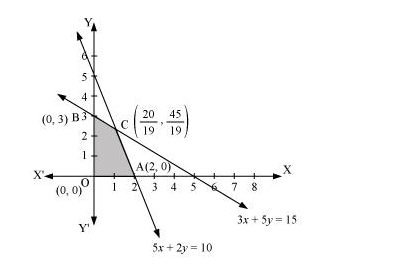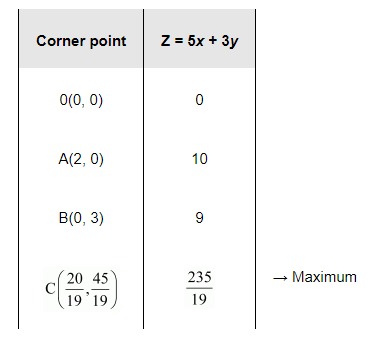# Maximise

Question:

Maximise Z = 5x + 3y

subject to $3 x+5 y \leq 15,5 x+2 y \leq 10, x \geq 0, y \geq 0$.

Solution:

The feasible region determined by the system of constraints, $3 x+5 y \leq 15$,

$5 x+2 y \leq 10, x \geq 0$, and $y \geq 0$, are as follows.The corner points of the feasible region are $\mathrm{O}(0,0), \mathrm{A}(2,0), \mathrm{B}(0,3)$, and $\mathrm{C}\left(\frac{20}{19}, \frac{45}{19}\right)$.

The values of Z at these corner points are as follows.Therefore, the maximum value of $Z$ is $\frac{235}{19}$ at the point $\left(\frac{20}{19}, \frac{45}{19}\right)$.